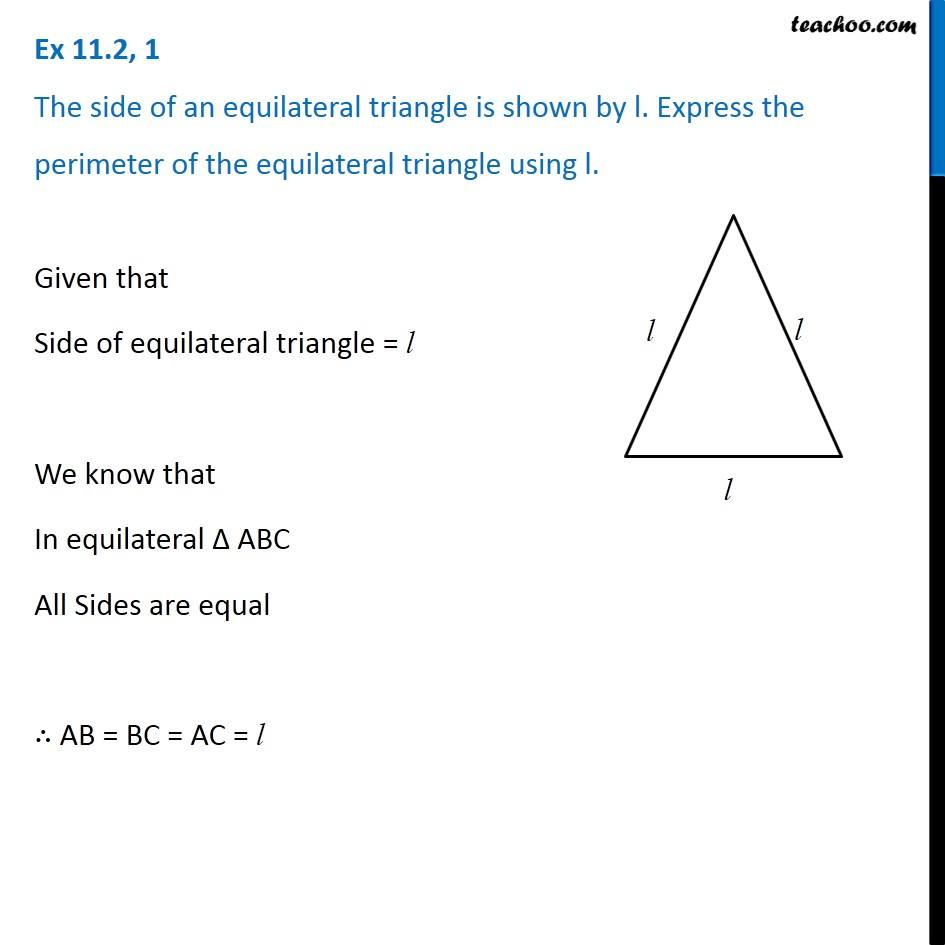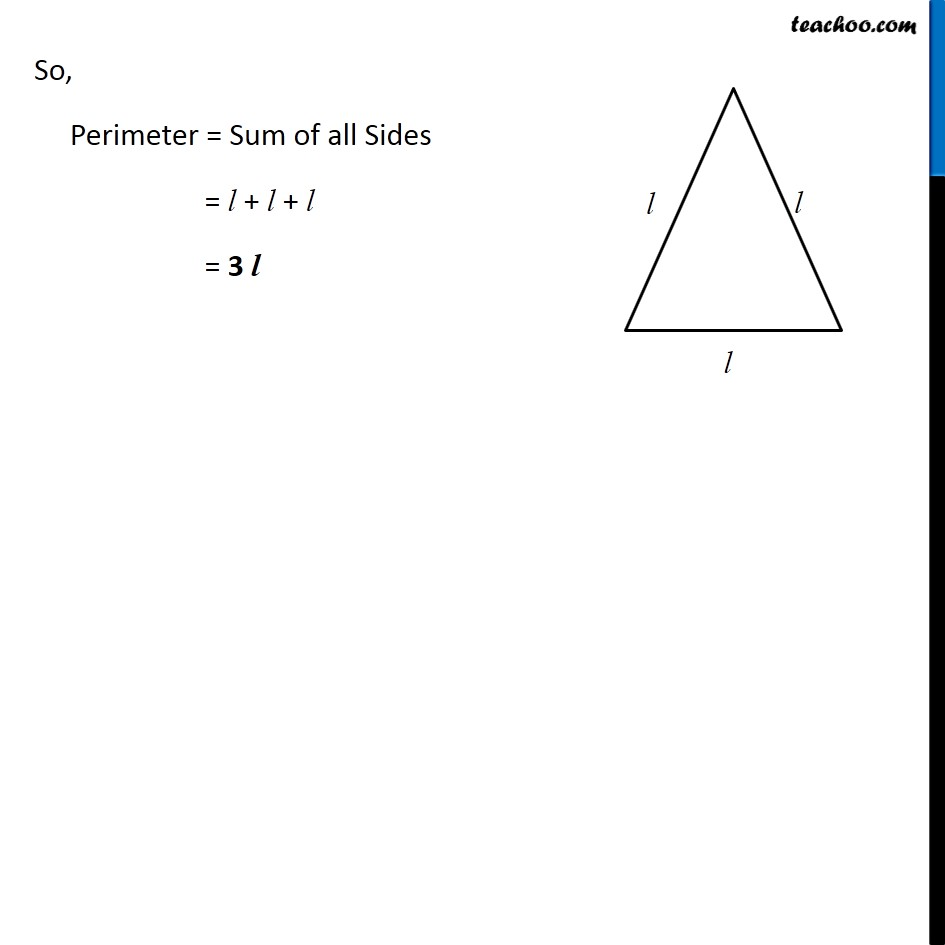Creating Algebra Equations using Geometry - Worksheet

Chapter 11 Class 6 Algebra
Serial order wiseLearn in your speed, with individual attention - Teachoo Maths 1-on-1 Class

### Transcript

Question 1 The side of an equilateral triangle is shown by l. Express the perimeter of the equilateral triangle using l. Given that Side of equilateral triangle = l We know that In equilateral ∆ ABC All Sides are equal ∴ AB = BC = AC = l So, Perimeter = Sum of all Sides = l + l + l = 3 l